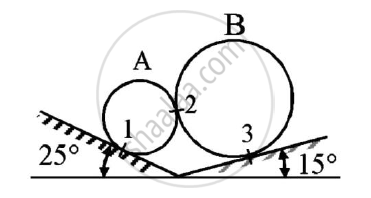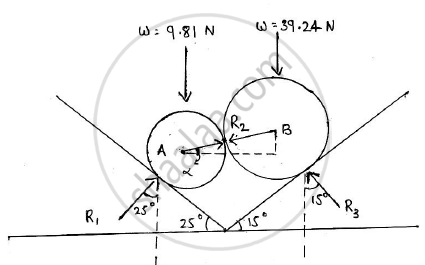# Determine the Reaction at Points of Constant 1,2 and 3. Assume Smooth Surfaces. - Engineering Mechanics

Determine the reaction at points of constant 1,2 and 3. Assume smooth surfaces.Given: The spheres are in equilibrium
To find: Reactions at points 1,2 and 3#### Solution

Considering both the spheres as a single body
The system of two spheres is in equilibrium
Applying conditions of equilibrium:
ΣFy=0
R1cos25 + R3cos15 – g - 4g = 0
R1cos25 + R3cos15 = 5g ……(1)
ΣFx = 0
R1sin25 - R3sin15 = 0 …….(2)
Solving (1) and (2)
R1= 19.75 N and R2 = 32.2493 N …….(3)
Let the reaction force between the wo spheres be R2 and it acts at an angle α with X-axis
Sphere A is in equilibrium
Applying conditions of equilibrium
ΣFy=0
R1cos25 - R2sinα – g = 0
R2sinα = 8.0896 ……..(4) (From 3)
ΣFx=0
R1sin25 - R2cosα = 0
R2cosα = 19.75sin25
R2cosα = 8.3467 ………(5)
Squaring and adding (4) and (5)
R22(cos2α+sin2α) = 135.1095
R2=11.6237 N
Dividing (4) by (5)
(R_2sinalpha)/(R_2cosalpha)=8.0896/8.3467
α = tan-1(0.9692)
=44.1038o
R1=19.75 N (75o with positive direction of X-axis in first quadrant)
R2=11.6237 N (44.1038o with negative direction of X-axis in third quadrant)
R3=32.2493 N (75o with negative direction of X axis in second quadrant)

Concept: Condition of Equilibrium for non-concurrent nonparallel general forces
Is there an error in this question or solution?